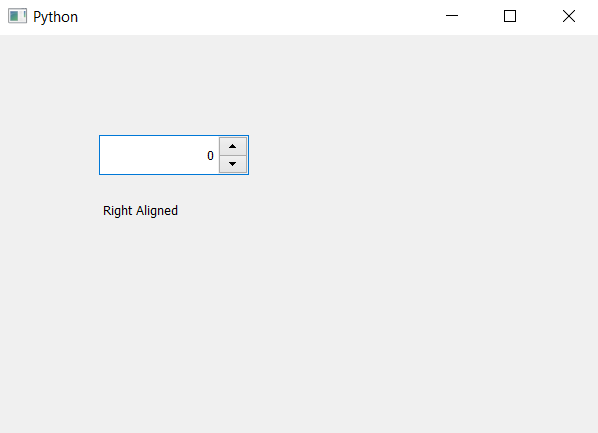Open In App

# PyQt5 QSpinBox – Checking if value is at right side

In this article we will see how we can check if the value appear in the right side of spin box or not, when we create spin box the value is at the left hand side in order to make the value in right we have to set its alignment flag to right. In order to do this we will use alignment method.

Syntax : spin_box.alignment()

Argument : It takes no argument

Return : It returns Qt object

Steps for implementation –

1. Create a spin box

2. Set its alignment with the help of setAlignment method

3. Create label to show the result

4. Get the alignment with the help of alignment method

5. Check if alignment is right then update the label

6. Show alignment is not right hand side

Below is the implementation

## Python3

 `# importing libraries``from` `PyQt5.QtWidgets ``import` `*``from` `PyQt5 ``import` `QtCore, QtGui``from` `PyQt5.QtGui ``import` `*``from` `PyQt5.QtCore ``import` `*``import` `sys` `class` `Window(QMainWindow):` `    ``def` `__init__(``self``):``        ``super``().__init__()` `        ``# setting title``        ``self``.setWindowTitle("Python ")` `        ``# setting geometry``        ``self``.setGeometry(``100``, ``100``, ``600``, ``400``)` `        ``# calling method``        ``self``.UiComponents()` `        ``# showing all the widgets``        ``self``.show()` `    ``# method for widgets``    ``def` `UiComponents(``self``):` `        ``# creating spin box``        ``self``.spin ``=` `QSpinBox(``self``)` `        ``# setting geometry to spin box``        ``self``.spin.setGeometry(``100``, ``100``, ``150``, ``40``)` `        ``# alignment``        ``alignment ``=` `Qt.AlignRight` `        ``# setting alignment``        ``self``.spin.setAlignment(alignment)` `        ``# creating label``        ``label ``=` `QLabel(``self``)` `        ``# setting geometry to the label``        ``label.setGeometry(``100``, ``160``, ``200``, ``30``)` `        ``# getting alignment``        ``get_alignment ``=` `self``.spin.alignment()` `        ``# checking if alignment is right``        ``if` `get_alignment ``=``=` `Qt.AlignRight:``            ``text ``=``" Right Aligned"` `        ``else``:``            ``text ``=``"Not right aligned"` `        ``# setting text to the label``        ``label.setText(text)`    `# create pyqt5 app``App ``=` `QApplication(sys.argv)` `# create the instance of our Window``window ``=` `Window()` `# start the app``sys.exit(App.``exec``())`

Output :